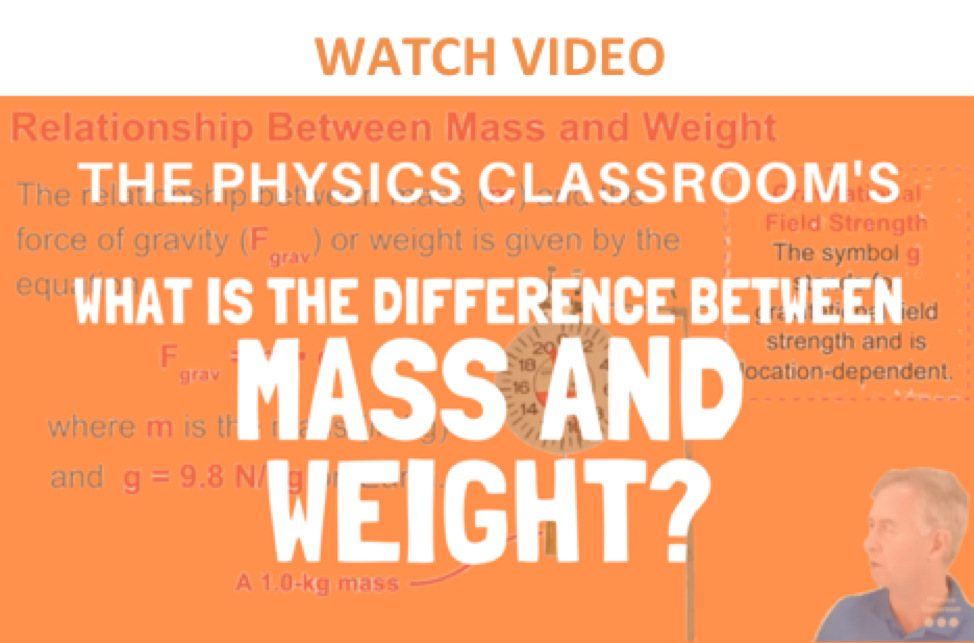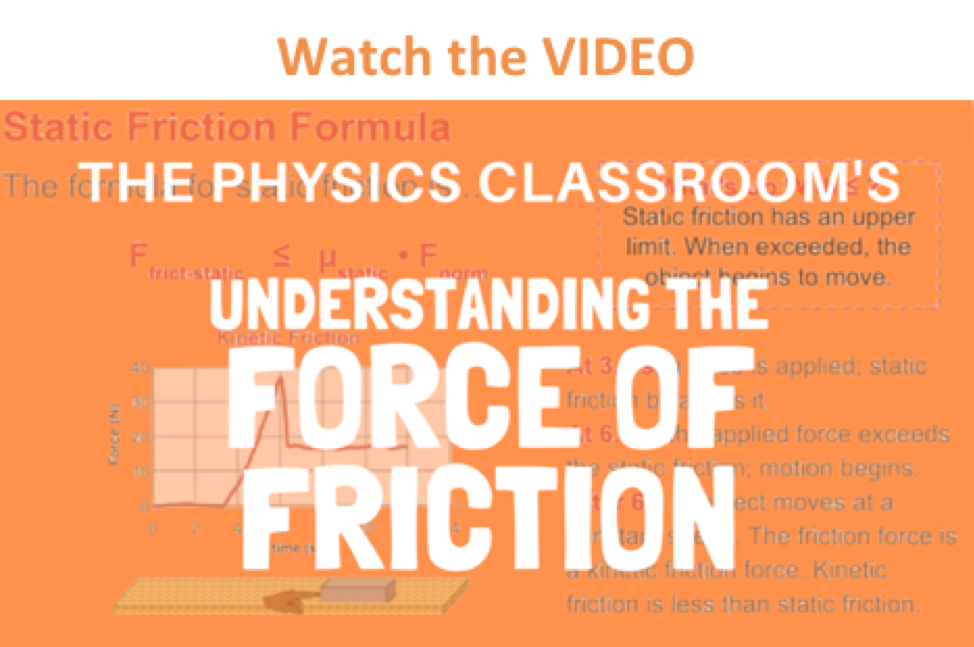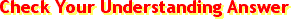Newton's Laws - Lesson 2 - Force and Its Representation

Types of Forces

A force is a push or pull acting upon an object as a result of its interaction with another object. There are a variety of types of forces. Previously in this lesson, a variety of force types were placed into two broad category headings on the basis of whether the force resulted from the contact or non-contact of the two interacting objects.

 Contact Forces Action-at-a-Distance Forces Frictional Force Gravitational Force Tension Force Electrical Force Normal Force Magnetic Force Air Resistance Force Applied Force Spring ForceThese types of individual forces will now be discussed in more detail. To read about each force listed above, continue scrolling through this page. Or to read about an individual force, click on its name from the list below.

Confusion of Mass and WeightA few further comments should be added about the single force that is a source of much confusion to many students of physics - the force of gravity. As mentioned above, the force of gravity acting upon an object is sometimes referred to as the weight of the object. Many students of physics confuse weight with mass. The mass of an object refers to the amount of matter that is contained by the object; the weight of an object is the force of gravity acting upon that object. Mass is related to how much stuff is there and weight is related to the pull of the Earth (or any other planet) upon that stuff. The mass of an object (measured in kg) will be the same no matter where in the universe that object is located. Mass is never altered by location, the pull of gravity, speed or even the existence of other forces. For example, a 2-kg object will have a mass of 2 kg whether it is located on Earth, the moon, or Jupiter; its mass will be 2 kg whether it is moving or not (at least for purposes of our study); and its mass will be 2 kg whether it is being pushed upon or not.

On the other hand, the weight of an object (measured in Newton) will vary according to where in the universe the object is. Weight depends upon which planet is exerting the force and the distance the object is from the planet. Weight, being equivalent to the force of gravity, is dependent upon the value of g - the gravitational field strength. On earth's surface g is 9.8 N/kg (often approximated as 10 N/kg). On the moon's surface, g is 1.7 N/kg. Go to another planet, and there will be another g value. Furthermore, the g value is inversely proportional to the distance from the center of the planet. So if we were to measure g at a distance of 400 km above the earth's surface, then we would find the g value to be less than 9.8 N/kg. (The nature of the force of gravity will be discussed in more detail in a later unit of The Physics Classroom.) Always be cautious of the distinction between mass and weight. It is the source of much confusion for many students of physics.

Flickr Physics Photo

A 1.0-kg mass is suspended from a spring scale in an effort to determine its weight. The scale reads just short of 10.0 N - close enough to call it 9.8 N. Mass refers to how much stuff is present in the object. Weight refers to the force with which gravity pulls upon the object.Investigate!

Even on the surface of the Earth, there are local variations in the value of g that have very small effects upon an object's weight. These variations are due to latitude, altitude and the local geological structure of the region. Use the Gravitational Fields widget below to investigate how location affects the value of g.

Sliding versus Static FrictionAs mentioned above, the friction force is the force exerted by a surface as an object moves across it or makes an effort to move across it. For the purpose of our study of physics at The Physics Classroom, there are two types of friction force - static friction and sliding friction. Sliding friction results when an object slides across a surface. As an example, consider pushing a box across a floor. The floor surface offers resistance to the movement of the box. We often say that the floor exerts a friction force upon the box. This is an example of a sliding friction force since it results from the sliding motion of the box. If a car slams on its brakes and skids to a stop (without antilock brakes), there is a sliding friction force exerted upon the car tires by the roadway surface. This friction force is also a sliding friction force because the car is sliding across the road surface. Sliding friction forces can be calculated from knowledge of the coefficient of friction and the normal force exerted upon the object by the surface it is sliding across. The formula is:

Ffrict-sliding = μfrict-sliding • Fnorm

The symbol μfrict-sliding represents the coefficient of sliding friction between the two surfaces. The coefficient value is dependent primarily upon the nature of the surfaces that are in contact with each other. For most surface combinations, the friction coefficients show little dependence upon other variables such as area of contact, temperature, etc. Values of μsliding have been experimentally determined for a variety of surface combinations and are often tabulated in technical manuals and handbooks. The values of μ provide a measure of the relative amount of adhesion or attraction of the two surfaces for each other. The more that surface molecules tend to adhere to each other, the greater the coefficient values and the greater the friction force.

Friction forces can also exist when the two surfaces are not sliding across each other. Such friction forces are referred to as static friction. Static friction results when the surfaces of two objects are at rest relative to one another and a force exists on one of the objects to set it into motion relative to the other object. Suppose you were to push with 5-Newton of force on a large box to move it across the floor. The box might remain in place. A static friction force exists between the surfaces of the floor and the box to prevent the box from being set into motion. The static friction force balances the force that you exert on the box such that the stationary box remains at rest. When exerting 5 Newton of applied force on the box, the static friction force has a magnitude of 5 Newton. Suppose that you were to push with 25 Newton of force on the large box and the box were to still remain in place. Static friction now has a magnitude of 25 Newton. Then suppose that you were to increase the force to 26 Newton and the box finally budged from its resting position and was set into motion across the floor. The box-floor surfaces were able to provide up to 25 Newton of static friction force to match your applied force. Yet the two surfaces were not able to provide 26 Newton of static friction force. The amount of static friction resulting from the adhesion of any two surfaces has an upper limit. In this case, the static friction force spans the range from 0 Newton (if there is no force upon the box) to 25 Newton (if you push on the box with 25 Newton of force). This relationship is often expressed as follows:

Ffrict-static ≤ μfrict-static• Fnorm

The symbol μfrict-static represents the coefficient of static friction between the two surfaces. Like the coefficient of sliding friction, this coefficient is dependent upon the types of surfaces that are attempting to move across each other. In general, values of static friction coefficients are greater than the values of sliding friction coefficients for the same two surfaces. Thus, it typically takes more force to budge an object into motion than it does to maintain the motion once it has been started.

The meaning of each of these forces listed in the table above will have to be thoroughly understood to be successful during this unit. Ultimately, you must be able to read a verbal description of a physical situation and know enough about these forces to recognize their presence (or absence) and to construct a free-body diagram that illustrates their relative magnitude and direction.

We Would Like to Suggest ...Sometimes it isn't enough to just read about it. You have to interact with it! And that's exactly what you do when you use one of The Physics Classroom's Interactives. We would like to suggest that you combine the reading of this page with the use of our Free-Body Diagram Interactive. You can find it in the Physics Interactives section of our website. The Free-Body Diagram Interactive allows a learner to practice identifying the forces that act upon an object and to express such an understanding by the construction of a free-body diagram.

1. Complete the following table showing the relationship between mass and weight.

 Object Mass (kg) Weight (N) Melon 1 kg See Answer9.8 N (or ~10 N if approximating g to be 10 m/s/s) Apple See Answer0.1 kg (or ~0.098 kg if approximating g to be 10 m/s/s) 0.98 N Pat Eatladee 25 kg See Answer245 N (or ~250 N if approximating g to be 10 m/s/s) Fred See Answer100 kg (or ~98 kg if approximating g to be 10 m/s/s) 980 N

2. Different masses are hung on a spring scale calibrated in Newtons.

1. The force exerted by gravity on 1 kg = 9.8 N.
2. The force exerted by gravity on 5 kg = ______ N.
3. The force exerted by gravity on _______ kg = 98 N.
4. The force exerted by gravity on 70 kg = ________ N.

3. When a person diets, is their goal to lose mass or to lose weight? Explain.

Next Section: